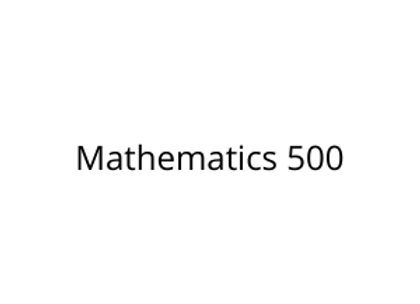# Mathematics 500

\$395.00Price
`Math 500 is a full-year elementary math course focusing on number skills, mathematical literacy, and geometric concepts. Students will gain solid experience with number theory and operations, including whole numbers, decimals, and fractions. In addition, students will develop their understanding of measurement and two- and three- dimensional figures. This course also integrates mathematical practices throughout the units, as well as introducing students to algebraic, statistical, and probability concepts.`